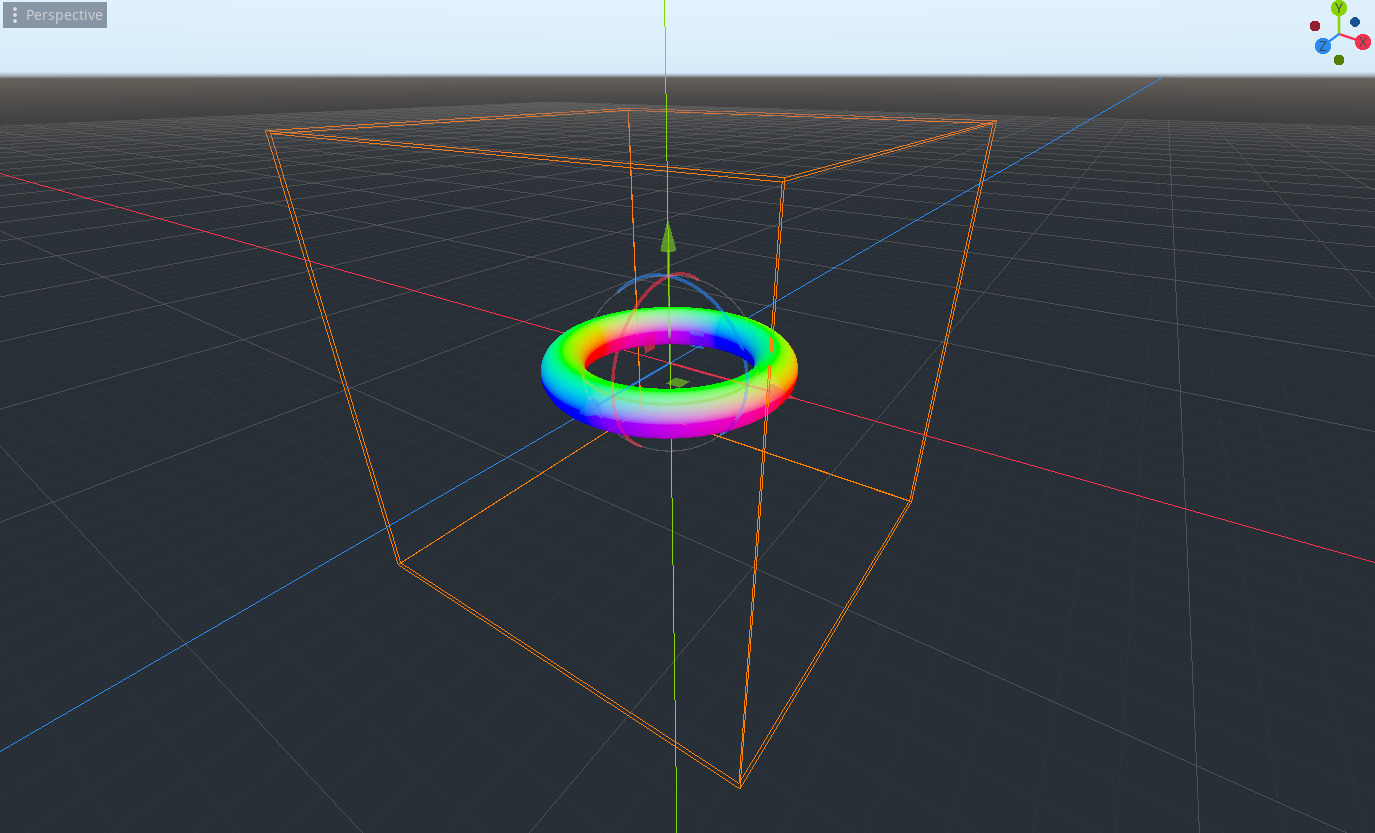# Raymarch in a box

This is simply a port of the “Writing a ray marcher in Unity” tutorial by The Art of Code. The world space to object space conversion wasn´t obvious, so hopefully this can be useful.

To revert back to world space, i.e. the “portal mode” that he mentions in the video, uncomment line 51 and set the render_mode to world_vertex_coords.

``````shader_type spatial;
//render_mode unshaded, world_vertex_coords; // to raymarch in world space

varying vec3 world_camera;
varying vec3 world_position;

const int MAX_STEPS = 100;
const float MAX_DIST = 100.0;
const float SURF_DIST = 1e-3;

float GetDist(vec3 p){
float d = length(p) - .5; //Sphere

d = length(vec2(length(p.xz) - .5, p.y)) - .1; //torus

return d;
}

float RayMarch(vec3 ro, vec3 rd) {
float dO = 0.0;
float dS;

for (int i = 0; i < MAX_STEPS; i++)
{
vec3 p = ro + dO * rd;
dS = GetDist(p);
dO += dS;

if (dS < SURF_DIST || dO > MAX_DIST)
break;
}
return dO;
}

vec3 GetNormal(vec3 p) {
vec2 e = vec2(1e-2, 0);

vec3 n = GetDist(p) - vec3(
GetDist(p - e.xyy),
GetDist(p - e.yxy),
GetDist(p - e.yyx)
);

return normalize(n);
}

void vertex() {
world_position = VERTEX;
world_camera = (inverse(MODELVIEW_MATRIX) * vec4(0, 0, 0, 1)).xyz; //object space
//world_camera = ( CAMERA_MATRIX  * vec4(0, 0, 0, 1)).xyz; //uncomment this to raymarch in world space
}

void fragment() {

vec3 ro = world_camera;
vec3 rd =  normalize(world_position - ro);

vec3 col;

float d = RayMarch(ro, rd);

if (d >= MAX_DIST)
else
{
vec3 p = ro + rd * d;
vec3 n = GetNormal(p);
col = n.rgb;
}

ALBEDO = col;
}``````
###### Tags
raymarchThe shader code and all code snippets in this post are under CC0 license and can be used freely without the author's permission. Images and videos, and assets depicted in those, do not fall under this license. For more info, see our License terms.

## More from kilojool

### Ray-box setup

Subscribe
Notify ofInline FeedbacksSRC Corp
2 years ago

thx! I can use this for a 4D game!SRC Corp
2 years ago

Jelle Venderme(I think thats his name) did a 4D game that uses raymarching.
I tried something like that myself but the system i ended up with was on a ColorRect. The 3D raymarching that you made can help me out in this 😀SRC Corp
2 years ago